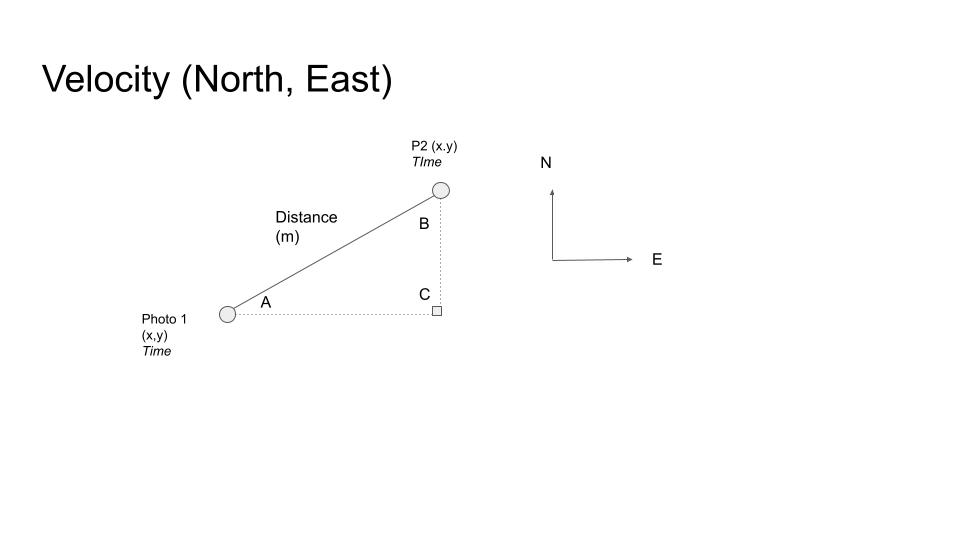Speed = distance / time. But what about speed along the x (east, west), y (north, south), z (altitude) axes?

Over the last few weeks I’ve been digging into creating and injecting CAMM telemetry from videos created from static frames.

The CAMM spec requires, in addition to latitude, longitude, and altitude:

• Velocity North (m/s)
• Velocity East
• Velocity Up

For the old school followers of our work, you might remember our old CLI tool, Sequence Maker.

This generated speed between photos as well as a whole host of other values like photo heading. This post explains everything.

Speed in this example was simplistically calculated (speed = distance/time) from point A to point B (the photo co-ordinates) along a 2D plane (no concept of the vertical axis).

However, for the CAMM values, I needed to convert speed into velocity… which is not the same as speed, as my old physics teacher would protest; velocity is speed with a direction.We already know the angles inside the right angle triangle (from working out heading).

Thus, we can use a bit of trigonometry to work out all lengths of the triangle (in addition from distance from A to B, which we already know).

We know `Velocity = Displacement / Time in a direction.`

So `Velocity East = Distance (along latitude) / Time (between source and destination)`

Or using the diagram above `Velocity East = Distance (from A to C) / Time (from A to B)`

And `Velocity North = Distance (along longitude) / Time (between source and destination)`

Or using the diagram above `Velocity North = Distance from C to B) / Time (from A to B)`

Note, this calculation can result in a negative output. North and East are positive directions. If you travel West/South, in terms of an East/North vector, you will be traveling in both a negative East/North velocity.

To illustrate the point; If I drive from home to work (defining my positive direction), then my velocity is positive if I go to work, but negative when I go home from work. It is all about direction seen from how I defined my positive axis.Look at this example to demonstrate. Whilst the time between A and B is really representative of 10 second / 10 metres, the North and Eastern velocity is actualy negative.

`Velocity East = Distance (from A to C) / Time (from A to B)`

so

`Velocity East = -5 / 10 = -0.5 m/s`

and

`Velocity North = Distance from C to B) / Time (from A to B)`

`Velocity North = -8.66025 / 10 = -0.8 m/s`

Now need to to the same for vertical velocity. It’s done in exactly the same way, except we now use the z axis (altitude).

So `Velocity Up = Distance (along vertical) / Time (between source and destination)`

So `Velocity Up = Elevation change (from A to B) / Time (from A to B)`

Again, if the elevation change is negative, the resulting Up velocity will be negative (because you’re descending).And that’s enough maths for a Friday… or whenever you’re reading this!